# Selina Solutions Concise Maths Class 7 Chapter 20: Mensuration (Perimeter and Area of Plane Figures) Exercise 20A

Selina Solutions Concise Maths Class 7 Chapter 20 Mensuration (Perimeter and Area of Plane Figures) Exercise 20A are available in PDF format to boost the exam preparation of students. The method of finding the perimeter of rectangle, square, triangle and circle are the concepts, which are discussed under this exercise. Various solved examples are present to help students understand the method of solving problems effortlessly. Students can access Selina Solutions Concise Maths Class 7 Chapter 20 Mensuration (Perimeter and Area of Plane Figures) Exercise 20A PDF, from the links provided here.

## Selina Solutions Concise Maths Class 7 Chapter 20: Mensuration (Perimeter and Area of Plane Figures) Exercise 20A Download PDF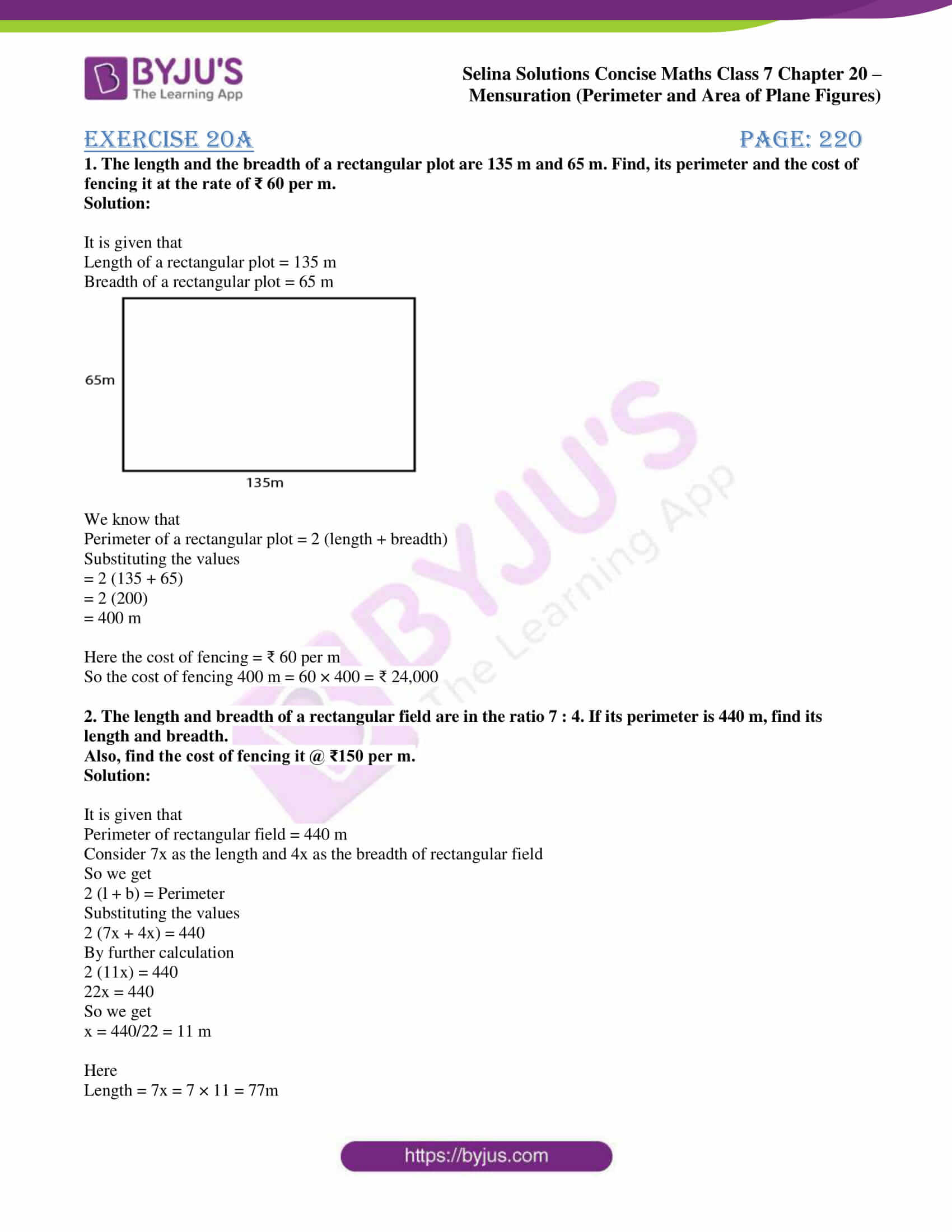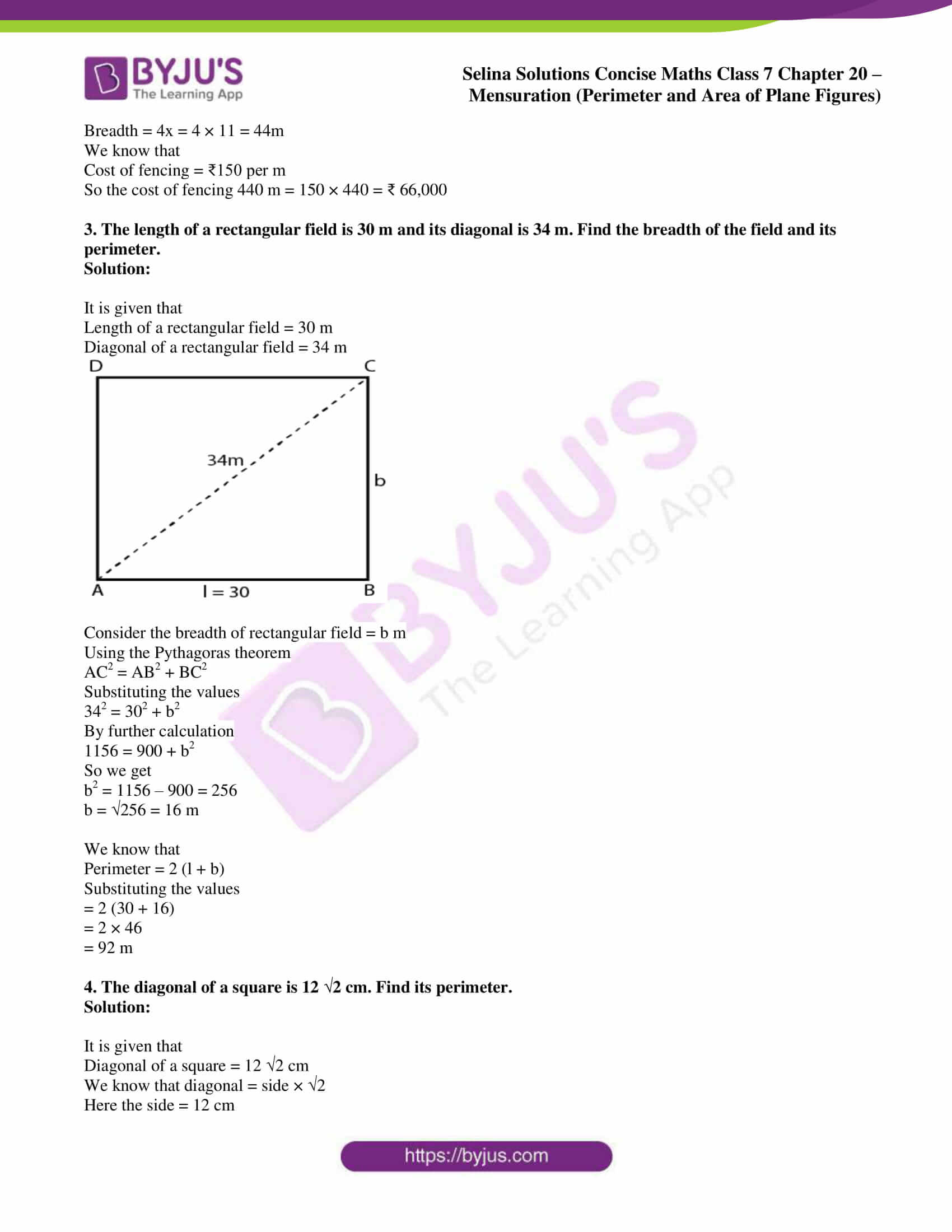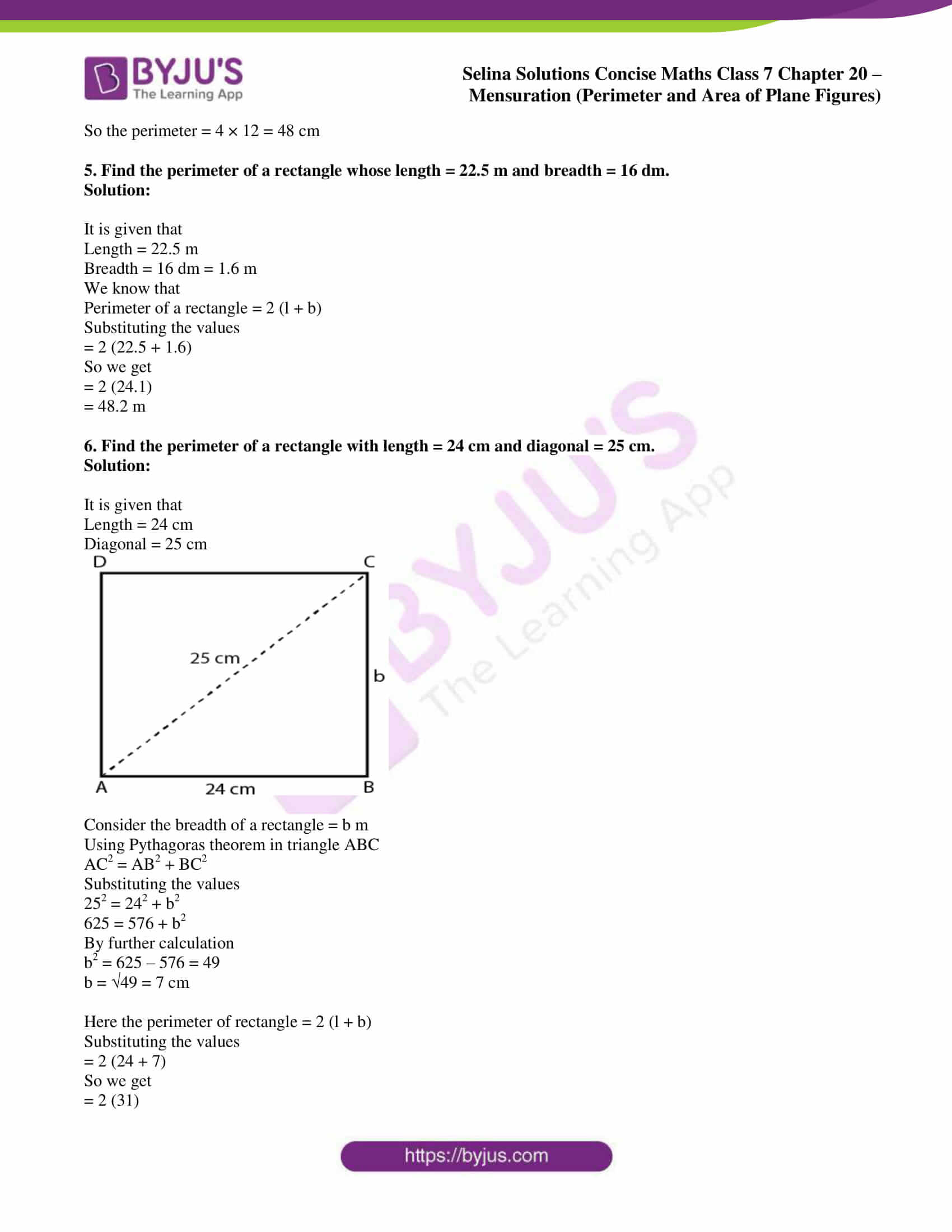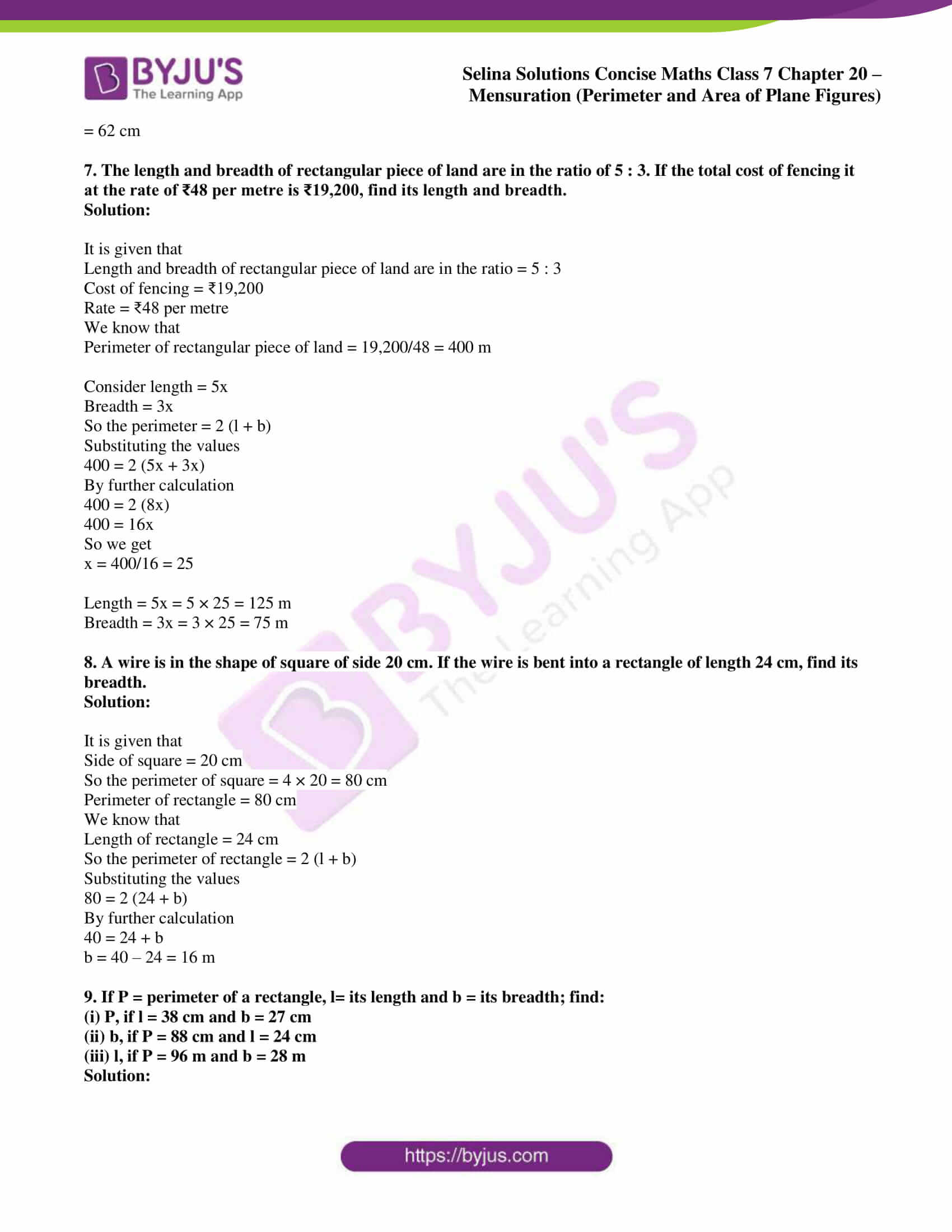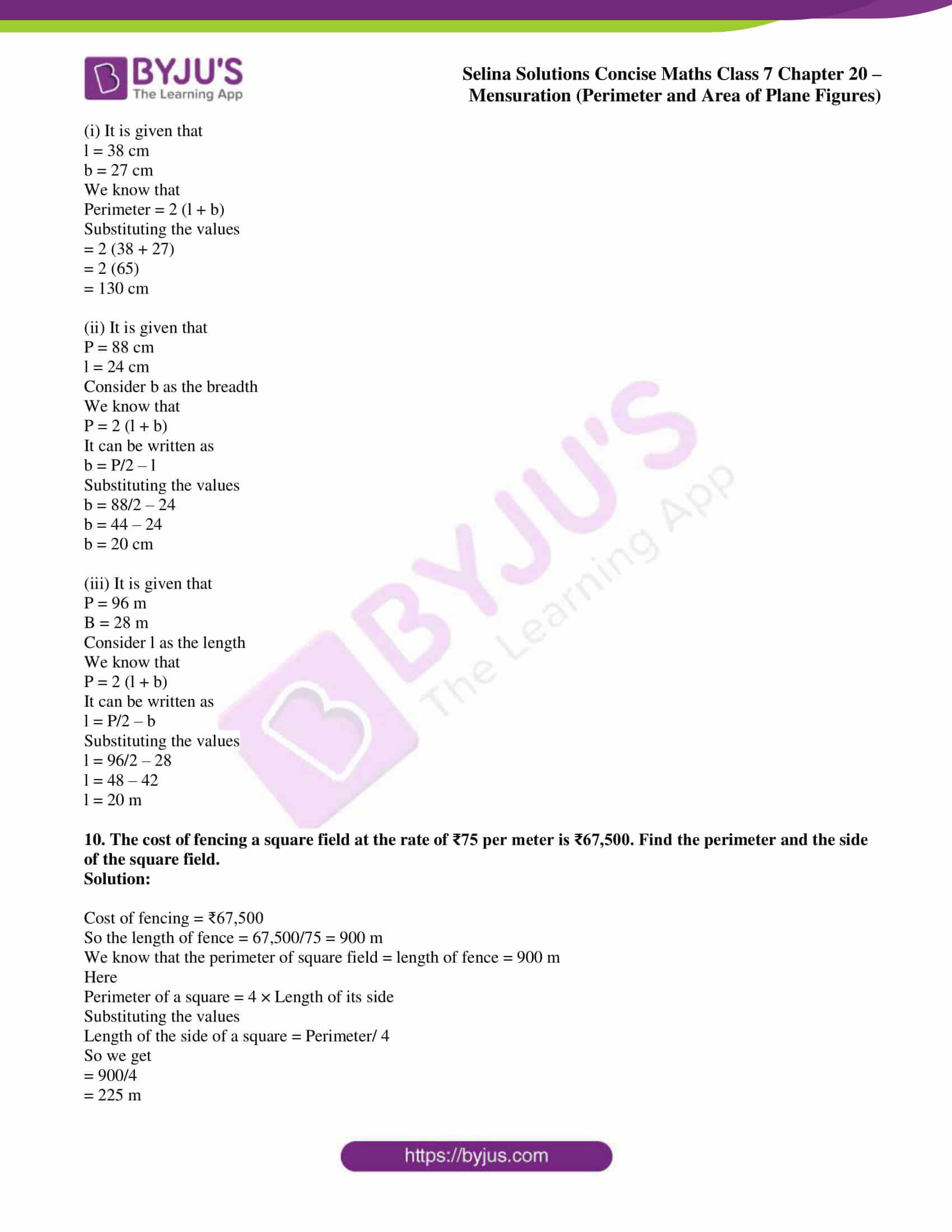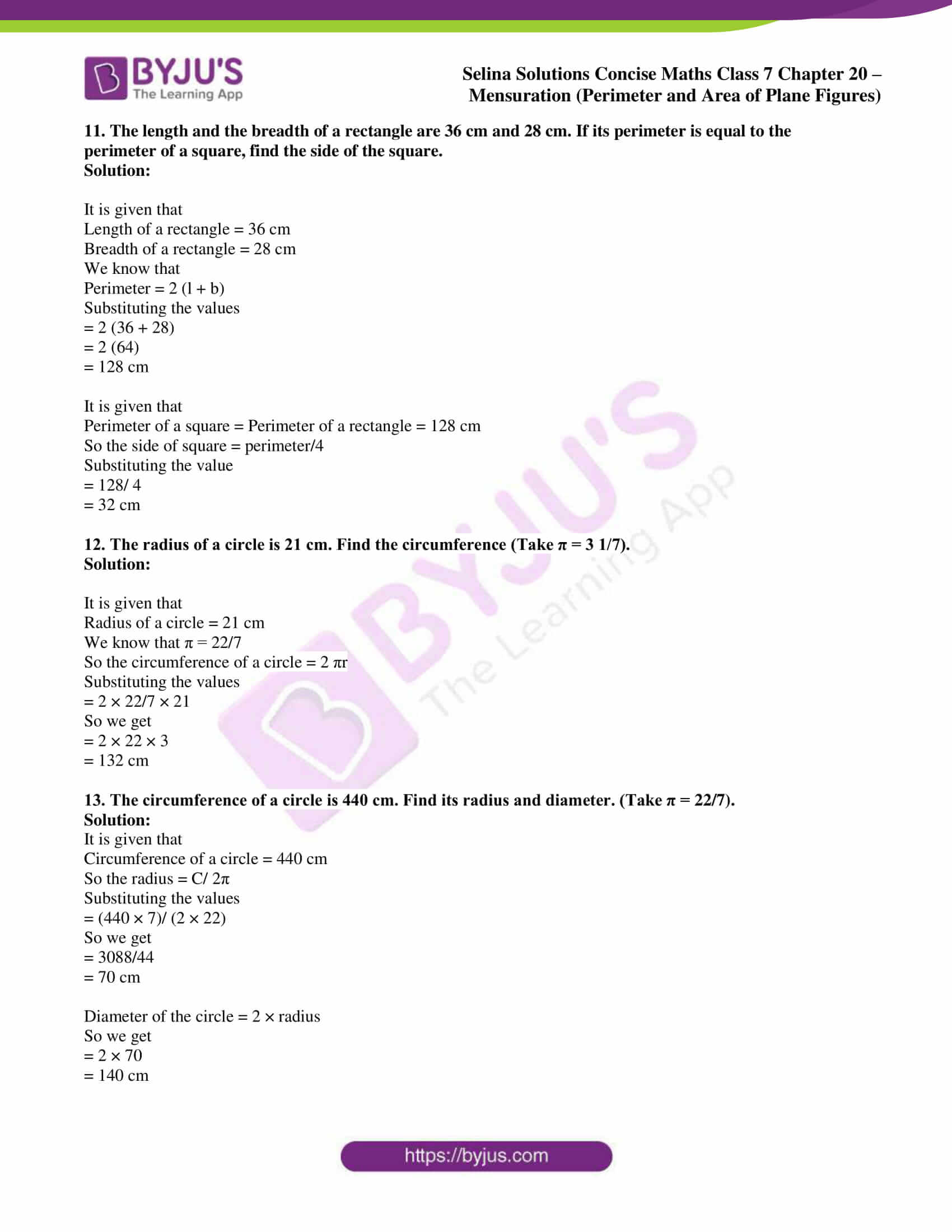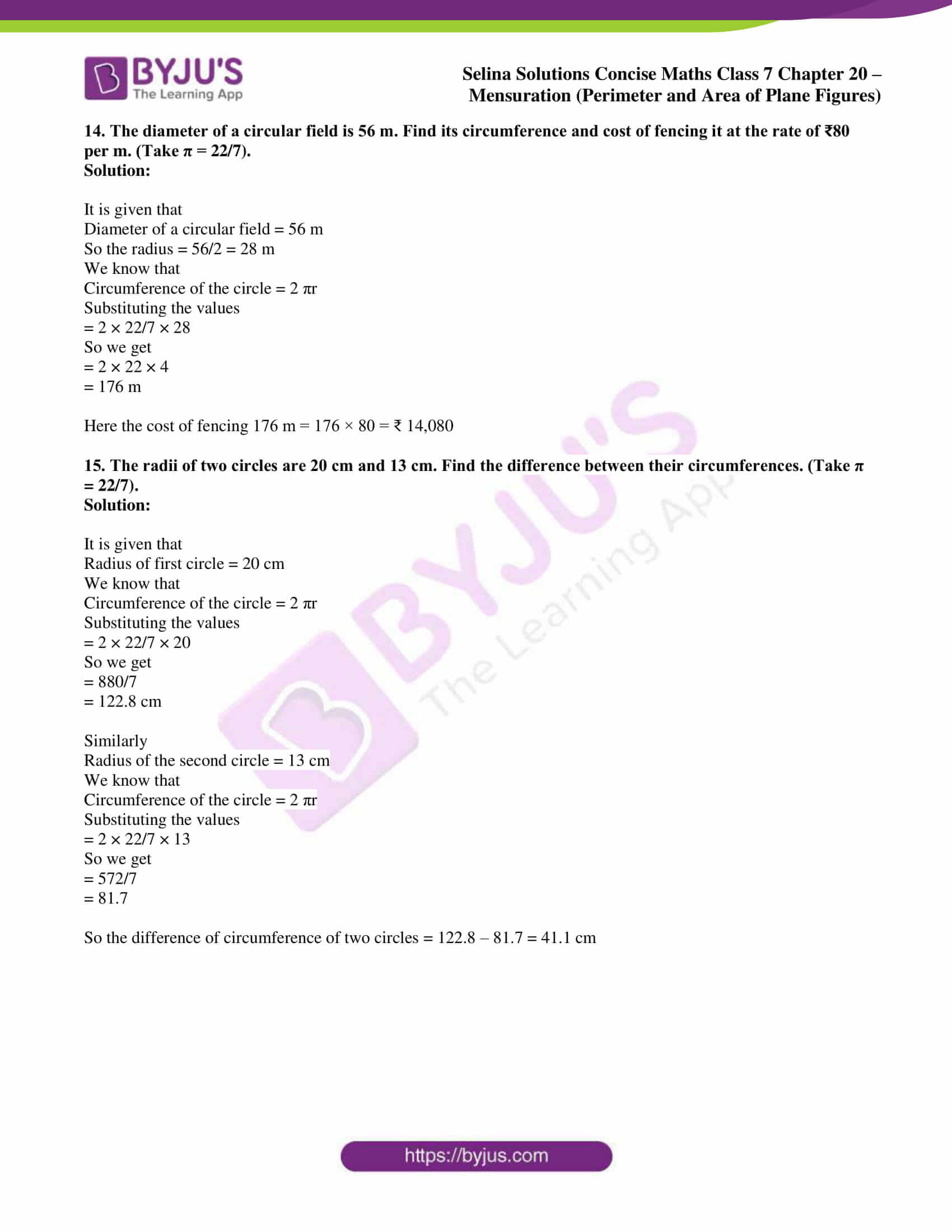### Access Selina Solutions Concise Maths Class 7 Chapter 20: Mensuration (Perimeter and Area of Plane Figures) Exercise 20A

#### Exercise 20A page: 220

1. The length and the breadth of a rectangular plot are 135 m and 65 m. Find, its perimeter and the cost of fencing it at the rate of ₹ 60 per m.

Solution:

It is given that

Length of a rectangular plot = 135 m

Breadth of a rectangular plot = 65 m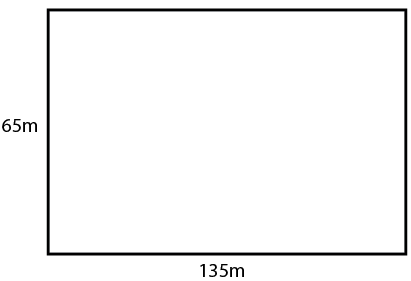We know that

Perimeter of a rectangular plot = 2 (length + breadth)

Substituting the values

= 2 (135 + 65)

= 2 (200)

= 400 m

Here the cost of fencing = ₹ 60 per m

So the cost of fencing 400 m = 60 × 400 = ₹ 24,000

2. The length and breadth of a rectangular field are in the ratio 7 : 4. If its perimeter is 440 m, find its length and breadth.

Also, find the cost of fencing it @ ₹150 per m.

Solution:

It is given that

Perimeter of rectangular field = 440 m

Consider 7x as the length and 4x as the breadth of rectangular field

So we get

2 (l + b) = Perimeter

Substituting the values

2 (7x + 4x) = 440

By further calculation

2 (11x) = 440

22x = 440

So we get

x = 440/22 = 11 m

Here

Length = 7x = 7 × 11 = 77m

Breadth = 4x = 4 × 11 = 44m

We know that

Cost of fencing = ₹150 per m

So the cost of fencing 440 m = 150 × 440 = ₹ 66,000

3. The length of a rectangular field is 30 m and its diagonal is 34 m. Find the breadth of the field and its perimeter.

Solution:

It is given that

Length of a rectangular field = 30 m

Diagonal of a rectangular field = 34 m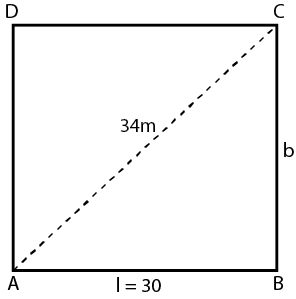Consider the breadth of rectangular field = b m

Using the Pythagoras theorem

AC2 = AB2 + BC2

Substituting the values

342 = 302 + b2

By further calculation

1156 = 900 + b2

So we get

b2 = 1156 – 900 = 256

b = √256 = 16 m

We know that

Perimeter = 2 (l + b)

Substituting the values

= 2 (30 + 16)

= 2 × 46

= 92 m

4. The diagonal of a square is 12 2 cm. Find its perimeter.

Solution:

It is given that

Diagonal of a square = 12 2 cm

We know that diagonal = side × √2

Here the side = 12 cm

So the perimeter = 4 × 12 = 48 cm

5. Find the perimeter of a rectangle whose length = 22.5 m and breadth = 16 dm.

Solution:

It is given that

Length = 22.5 m

Breadth = 16 dm = 1.6 m

We know that

Perimeter of a rectangle = 2 (l + b)

Substituting the values

= 2 (22.5 + 1.6)

So we get

= 2 (24.1)

= 48.2 m

6. Find the perimeter of a rectangle with length = 24 cm and diagonal = 25 cm.

Solution:

It is given that

Length = 24 cm

Diagonal = 25 cm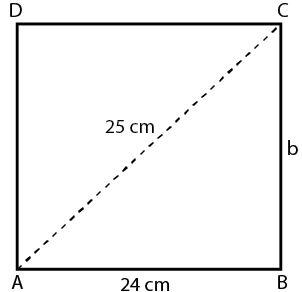Consider the breadth of a rectangle = b m

Using Pythagoras theorem in triangle ABC

AC2 = AB2 + BC2

Substituting the values

252 = 242 + b2

625 = 576 + b2

By further calculation

b2 = 625 – 576 = 49

b = √49 = 7 cm

Here the perimeter of rectangle = 2 (l + b)

Substituting the values

= 2 (24 + 7)

So we get

= 2 (31)

= 62 cm

7. The length and breadth of rectangular piece of land are in the ratio of 5 : 3. If the total cost of fencing it at the rate of ₹48 per metre is ₹19,200, find its length and breadth.

Solution:

It is given that

Length and breadth of rectangular piece of land are in the ratio = 5 : 3

Cost of fencing = ₹19,200

Rate = ₹48 per metre

We know that

Perimeter of rectangular piece of land = 19,200/48 = 400 m

Consider length = 5x

So the perimeter = 2 (l + b)

Substituting the values

400 = 2 (5x + 3x)

By further calculation

400 = 2 (8x)

400 = 16x

So we get

x = 400/16 = 25

Length = 5x = 5 × 25 = 125 m

Breadth = 3x = 3 × 25 = 75 m

8. A wire is in the shape of square of side 20 cm. If the wire is bent into a rectangle of length 24 cm, find its breadth.

Solution:

It is given that

Side of square = 20 cm

So the perimeter of square = 4 × 20 = 80 cm

Perimeter of rectangle = 80 cm

We know that

Length of rectangle = 24 cm

So the perimeter of rectangle = 2 (l + b)

Substituting the values

80 = 2 (24 + b)

By further calculation

40 = 24 + b

b = 40 – 24 = 16 m

9. If P = perimeter of a rectangle, l= its length and b = its breadth; find:

(i) P, if l = 38 cm and b = 27 cm

(ii) b, if P = 88 cm and l = 24 cm

(iii) l, if P = 96 m and b = 28 m

Solution:

(i) It is given that

l = 38 cm

b = 27 cm

We know that

Perimeter = 2 (l + b)

Substituting the values

= 2 (38 + 27)

= 2 (65)

= 130 cm

(ii) It is given that

P = 88 cm

l = 24 cm

We know that

P = 2 (l + b)

It can be written as

b = P/2 – l

Substituting the values

b = 88/2 – 24

b = 44 – 24

b = 20 cm

(iii) It is given that

P = 96 m

B = 28 m

Consider l as the length

We know that

P = 2 (l + b)

It can be written as

l = P/2 – b

Substituting the values

l = 96/2 – 28

l = 48 – 42

l = 20 m

10. The cost of fencing a square field at the rate of ₹75 per meter is ₹67,500. Find the perimeter and the side of the square field.

Solution:

Cost of fencing = ₹67,500

So the length of fence = 67,500/75 = 900 m

We know that the perimeter of square field = length of fence = 900 m

Here

Perimeter of a square = 4 × Length of its side

Substituting the values

Length of the side of a square = Perimeter/ 4

So we get

= 900/4

= 225 m

11. The length and the breadth of a rectangle are 36 cm and 28 cm. If its perimeter is equal to the perimeter of a square, find the side of the square.

Solution:

It is given that

Length of a rectangle = 36 cm

Breadth of a rectangle = 28 cm

We know that

Perimeter = 2 (l + b)

Substituting the values

= 2 (36 + 28)

= 2 (64)

= 128 cm

It is given that

Perimeter of a square = Perimeter of a rectangle = 128 cm

So the side of square = perimeter/4

Substituting the value

= 128/ 4

= 32 cm

12. The radius of a circle is 21 cm. Find the circumference (Take π = 3 1/7).

Solution:

It is given that

Radius of a circle = 21 cm

We know that π = 22/7

So the circumference of a circle = 2 πr

Substituting the values

= 2 × 22/7 × 21

So we get

= 2 × 22 × 3

= 132 cm

13. The circumference of a circle is 440 cm. Find its radius and diameter. (Take π = 22/7).

Solution:

It is given that

Circumference of a circle = 440 cm

So the radius = C/ 2π

Substituting the values

= (440 × 7)/ (2 × 22)

So we get

= 3088/44

= 70 cm

Diameter of the circle = 2 × radius

So we get

= 2 × 70

= 140 cm

14. The diameter of a circular field is 56 m. Find its circumference and cost of fencing it at the rate of ₹80 per m. (Take π = 22/7).

Solution:

It is given that

Diameter of a circular field = 56 m

So the radius = 56/2 = 28 m

We know that

Circumference of the circle = 2 πr

Substituting the values

= 2 × 22/7 × 28

So we get

= 2 × 22 × 4

= 176 m

Here the cost of fencing 176 m = 176 × 80 = ₹ 14,080

15. The radii of two circles are 20 cm and 13 cm. Find the difference between their circumferences. (Take π = 22/7).

Solution:

It is given that

Radius of first circle = 20 cm

We know that

Circumference of the circle = 2 πr

Substituting the values

= 2 × 22/7 × 20

So we get

= 880/7

= 122.8 cm

Similarly

Radius of the second circle = 13 cm

We know that

Circumference of the circle = 2 πr

Substituting the values

= 2 × 22/7 × 13

So we get

= 572/7

= 81.7

So the difference of circumference of two circles = 122.8 – 81.7 = 41.1 cm